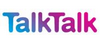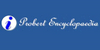### EpicycloidIn geometry, an epicycloid is a plane curve produced by tracing the path of a chosen point of a circle — called an epicycle — which rolls without slipping around a fixed circle. It is a particular kind of roulette. If k is an integer, then the curve is closed, and has k cusps (i.e., sharp corners, where the curve is not differentiable). If k i...
Found on http://en.wikipedia.org/wiki/Epicycloid

### Epicycloid• (n.) A curve traced by a point in the circumference of a circle which rolls on the convex side of a fixed circle.
Found on http://thinkexist.com/dictionary/meaning/epicycloid/

### epicycloid<geometry> A curve traced by a point in the circumference of a circle which rolls on the convex side of a fixed circle. ... Any point rigidly connected with the rolling circle, but not in its circumference, traces a curve called an epitrochoid. The curve traced by a point in the circumference of the rolling circle when it rolls on the concave...
Found on http://www.encyclo.co.uk/local/20973

### epicycloidnoun a line generated by a point on a circle rolling around another circle
Found on https://www.encyclo.co.uk/local/20974

### EpicycloidEp`i·cy'cloid noun [ Epicycle + -oid : confer French épicycloïde .] (Geom.) A curve traced by a point in the circumference of a circle which rolls on the convex side of a fixed circle. » Any point rigidly connected with the rolling circle, but not in its circumferen...
Found on http://www.encyclo.co.uk/webster/E/54

### epicycloidIn geometry, a curve resembling a series of arches traced out by a point on the circumference of a circle that rolls around another circle of a different diameter. If the two circles have the same diameter, the curve is a cardioid
Found on http://www.talktalk.co.uk/reference/encyclopaedia/hutchinson/m0006674.html

### EpicycloidIn geometry, an epicycloid is a curve generated by the movement of a circle upon the convex side of another curve, that generated by the movement of a circle upon the concave side of a fixed curve being called a hypocycloid.
Found on http://www.probertencyclopaedia.com/browse/GE.HTM

### epicycloidThe path traced out by a point on the circumference of a circle of radius b rolling on the outside of a circle of radius a. It is described by the parametric equations: x = (a + b) cos(t) - b cos[(a/b + 1)t], y = (a + b) sin(t...
Found on http://www.daviddarling.info/encyclopedia/E/epicycloid.html

### epicycloid[n] - a line generated by a point on a circle rolling around another circle
Found on http://www.webdictionary.co.uk/definition.php?query=epicycloid
No exact match found